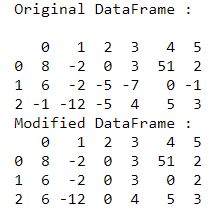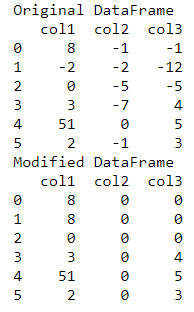# Replace negative values with latest preceding positive value in Pandas DataFrame

In this article, we will discuss how to replace the negative value in Pandas DataFrame Column with the latest preceding positive value.

While doing this there may arise two situations –

• Value remains unmodified if no proceeding positive value exists
• Value update to 0 if no proceeding positive value exists

Let’s discuss these cases in detail.

Case 1: Value remain unmodified if no proceeding positive value exists

A variable is declared to store the latest preceding positive value initialized with some large negative integer. An iteration of the data frame is then performed column-wise.

• In case the value is negative, it is replaced with the preceding value positive variable if it exists otherwise, it remains unmodified.
• And, in case the value is positive, the preceding positive value variable is updated.

Example:

## Python3

 `import` `pandas as pd ` ` `  ` `  `# creating a pandas dataframe ` `df ``=` `pd.DataFrame([[``8``, ``-``2``, ``0``, ``3``, ``51``, ``2``], ` `                   ``[``6``, ``-``2``, ``-``5``, ``-``7``, ``0``, ``-``1``], ` `                   ``[``-``1``, ``-``12``, ``-``5``, ``4``, ``5``, ``3``]]) ` `print``(``"Original DataFrame : \n"``) ` `print``(df) ` ` `  `# declaring a pre defined value ` `prec_val ``=` `-``999` ` `  `# iterate over columns ` `for` `i ``in` `range``(df.shape[``1``]): ` ` `  `    ``# resetting value over each column ` `    ``prec_val ``=` `-``999` ` `  `    ``# iterate over rows ` `    ``for` `j ``in` `range``(df.shape[``0``]): ` ` `  `        ``# accessing the cell value ` `        ``cell ``=` `df.at[j, i] ` ` `  `        ``# check if cell value is negative ` `        ``if``(cell < ``0``): ` ` `  `            ``# check if prec_val is not default ` `            ``# set value ` `            ``if``(prec_val !``=` `-``999``): ` ` `  `                ``# replace the cell value ` `                ``df.at[j, i] ``=` `prec_val ` `        ``else``: ` ` `  `            ``# store the latest value in variable ` `            ``prec_val ``=` `df.at[j, i] ` ` `  `print``(``"Modified DataFrame : "``) ` `print``(df) `

Output:Case 2: Value update to 0 if no proceeding positive value exists

This approach uses the concept of data frame masking in order to replace the negative values of the data frame. The values are traversed from left to right column-wise, in a top to bottom manner. In this approach, initially, all the values < 0 in the data frame cells are converted to NaN.

Pandas dataframe.ffill() method is used to fill the missing values in the data frame. ‘ffill’ in this method stands for ‘forward fill’ and it propagates the last valid encountered observation forward. The ffill() function is used to fill the missing values along the index axis that is specified. This method has the following syntax :

Syntax: DataFrame.ffill(axis=None, inplace=False)

Parameters:

• axis – {0, index 1, column}
• inplace : If True, fill in place.

This is followed by the fillna() method to fill the NA/NaN values using the specified value. Here, we fill the NaN values by 0, since it is the lowest positive integer value possible. All the negative values are thus converted to positive ones. This approach can work on data frames, that don’t have any string values stored. In case there are no preceding positive values, then the negative value is replaced by 0.

## Python3

 `import` `pandas as pd ` ` `  `# creating a pandas dataframe ` `data_frame ``=` `pd.DataFrame({``'col1'``: [``8``, ``-``2``, ``0``, ``3``, ``51``, ``2``], ` `                           ``'col2'``: [``-``1``, ``-``2``, ``-``5``, ``-``7``, ``0``, ``-``1``], ` `                           ``'col3'``: [``-``1``, ``-``12``, ``-``5``, ``4``, ``5``, ``3``]}) ` ` `  `print``(``"Original DataFrame"``) ` `print``(data_frame) ` ` `  `# masking the data frame ` `data_frame ``=` `data_frame.mask(data_frame.lt( ` `    ``0``)).ffill().fillna(``0``).astype(``'int32'``) ` ` `  `print``(``"Modified DataFrame"``) ` `print``(data_frame) `

Output:Whether you're preparing for your first job interview or aiming to upskill in this ever-evolving tech landscape, GeeksforGeeks Courses are your key to success. We provide top-quality content at affordable prices, all geared towards accelerating your growth in a time-bound manner. Join the millions we've already empowered, and we're here to do the same for you. Don't miss out - check it out now!

Previous
Next